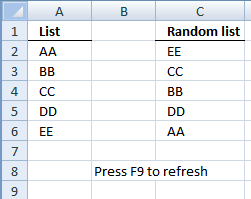Author: Oscar Cronquist Article last updated on April 06, 2011

### Overview

The array formula in cell range C2:C6 creates a random list from the values in cell range A2:A6.### Array formula in C2:

=INDEX(List, LARGE((ROW(List)-MIN(ROW(List))+1)*(COUNTIF(\$C\$1:C1, List)<>COUNTIF(List, List)), RANDBETWEEN(1, SUM(--(COUNTIF(\$C\$1:C1, List)<>COUNTIF(List, List)))))) + CTRL + SHIFT + ENTER.

### How to create an array formula

1. Copy (Ctrl + c) and paste (Ctrl + v) array formula into formula bar.
2. Press and hold Ctrl + Shift.
3. Press Enter once.
4. Release all keys.

Copy cell C2 and paste it down as far as needed.

### How this formula works in cell C2

Step 1 - Create an array of row numbers from the named range List

=INDEX(List, LARGE((ROW(List)-MIN(ROW(List))+1)*(COUNTIF(\$C\$1:C1, List)<>COUNTIF(List, List)), RANDBETWEEN(1, SUM(--(COUNTIF(\$C\$1:C1, List)<>COUNTIF(List, List))))))

ROW(reference) returns the rownumber of a reference

(ROW(List)-MIN(ROW(List))+1)

becomes

(ROW(A2:A6)-MIN(ROW(A2:A6))+1)

becomes

{2;3;4;5;6}-MIN(ROW({2;3;4;5;6}))+1

becomes

{2;3;4;5;6}-2+1

becomes

{1;2;3;4;5}

Step 2 - Check value frequency

=INDEX(List, LARGE((ROW(List)-MIN(ROW(List))+1)*(COUNTIF(\$C\$1:C1, List)<>COUNTIF(List, List)), RANDBETWEEN(1, SUM(--(COUNTIF(\$C\$1:C1, List)<>COUNTIF(List, List))))))

COUNTIF(range,criteria) counts the number of cells within a range that meet the given condition

(COUNTIF(\$C\$1:C1, List)<>COUNTIF(List, List))

becomes

(COUNTIF("Random list", {"AA";"DD";"CC";"DD";"EE"})<>COUNTIF(List, List))

becomes

{0;0;0;0;0}<>COUNTIF({"AA";"DD";"CC";"DD";"EE"}, {"AA";"DD";"CC";"DD";"EE"})

becomes

{0;0;0;0;0}<>{1;2;1;2;1}

and returns

{TRUE; TRUE; TRUE; TRUE; TRUE}

Step 3 - Calcultate randbetween arguments

=INDEX(List, LARGE((ROW(List)-MIN(ROW(List))+1)*(COUNTIF(\$C\$1:C1, List)<>COUNTIF(List, List)), RANDBETWEEN(1, SUM(--(COUNTIF(\$C\$1:C1, List)<>COUNTIF(List, List))))))

RANDBETWEEN(bottom, top) returns a random number between the numbers you specify.

RANDBETWEEN(1, SUM(--(COUNTIF(\$C\$1:C1, List)<>COUNTIF(List, List)))))

becomes

RANDBETWEEN(1, SUM(--({0;0;0;0;0}<>{1;2;1;2;1}))))

becomes

RANDBETWEEN(1, SUM(--({TRUE; TRUE; TRUE; TRUE; TRUE}))))

becomes

RANDBETWEEN(1, SUM({1; 1; 1; 1; 1})))

becomes

RANDBETWEEN(1, 5)

and returns a random number between 1 and 5.

Step 4 - Select a random row number

=INDEX(List, LARGE((ROW(List)-MIN(ROW(List))+1)*(COUNTIF(\$C\$1:C1, List)<>COUNTIF(List, List)), RANDBETWEEN(1, SUM(--(COUNTIF(\$C\$1:C1, List)<>COUNTIF(List, List))))))

LARGE(array,k) returns the k-th largest row number in this data set.

becomes

=INDEX(List,LARGE({1;2;3;4;5}, 1))

becomes

=INDEX({"AA";"DD";"CC";"DD";"EE"}, LARGE({1;2;3;4;5}, 1))

and returns EE in cell C2. (random)

Change the named ranges. If your random list starts at cell F2, change cell reference C1:\$C\$1 in the above formula to \$F\$1:F1.

### Named ranges

List (A2:A6)
What is named ranges?

### Get excel sample file for this tutorial.

sort a list in random order.xls
(Excel 97-2003 Workbook *.xls)

SUMPRODUCT(array1, array2, )
Returns the sum of the products of the corresponding ranges or arrays

COUNTIF(range,criteria)
Counts the number of cells within a range that meet the given condition

LARGE(array,k) returns the k-th largest row number in this data set.

ROW(reference) returns the rownumber of a reference

INDEX(array,row_num,[column_num])
Returns a value or reference of the cell at the intersection of a particular row and column, in a given range

RANDBETWEEN(bottom, top)
Returns a random number between the numbers you specify

ISERROR(value)
Checks whether a value is an error and returns TRUE or FALSE

MATCH(lookup_value;lookup_array; [match_type])
Returns the relative position of an item in an array that matches a specified value

MIN(number1,[number2])
Returns the smallest number in a set of values. Ignores logical values and text